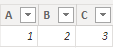cancel
Showing results for
Did you mean:Frequent Visitor

## How to add columns name as a row in new column?

I wan to show some column names as a row in new column.

1 ACCEPTED SOLUTIONCommunity Support

Hi  @Alice08 ,

I created some data:

Table:Here are the steps you can follow：

1. Create calculated column.

You can use NAMEOF() functions to get table and column names:

``NAMEOF('Table'[A])``You can use the Mid() function to intercept:

``````Column =
MID(
NAMEOF('Table'[A]),9,1) &""& MID( NAMEOF('Table'[B]),9,1)``````

2. Result:Best Regards,

Liu Yang

If this post helps, then please consider Accept it as the solution to help the other members find it more quickly

2 REPLIES 2Community Support

Hi  @Alice08 ,

I created some data:

Table:Here are the steps you can follow：

1. Create calculated column.

You can use NAMEOF() functions to get table and column names:

``NAMEOF('Table'[A])``You can use the Mid() function to intercept:

``````Column =
MID(
NAMEOF('Table'[A]),9,1) &""& MID( NAMEOF('Table'[B]),9,1)``````

2. Result:Best Regards,

Liu Yang

If this post helps, then please consider Accept it as the solution to help the other members find it more quicklySuper User

NewStep=Table.Unpivot(PreviousStepName,{"ColumnName"},"ColumnName","Value")